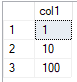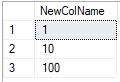# Quickstart: Run simple Python scripts with SQL machine learning

Applies to:SQL Server 2017 (14.x) and laterAzure SQL Managed Instance

In this quickstart, you'll run a set of simple Python scripts using SQL Server Machine Learning Services, Azure SQL Managed Instance Machine Learning Services, or SQL Server Big Data Clusters. You'll learn how to use the stored procedure sp_execute_external_script to execute the script in a SQL Server instance.

## Prerequisites

You need the following prerequisites to run this quickstart.

## Run a simple script

To run a Python script, you'll pass it as an argument to the system stored procedure, sp_execute_external_script. This system stored procedure starts the Python runtime in the context of SQL machine learning, passes data to Python, manages Python user sessions securely, and returns any results to the client.

In the following steps, you'll run this example Python script in your database:

``````a = 1
b = 2
c = a/b
d = a*b
print(c, d)
``````
1. Open a new query window in Azure Data Studio connected to your SQL instance.

2. Pass the complete Python script to the `sp_execute_external_script` stored procedure.

The script is passed through the `@script` argument. Everything inside the `@script` argument must be valid Python code.

``````EXECUTE sp_execute_external_script @language = N'Python'
, @script = N'
a = 1
b = 2
c = a/b
d = a*b
print(c, d)
'
``````
3. The correct result is calculated and the Python `print` function returns the result to the Messages window.

It should look something like this.

Results

``````STDOUT message(s) from external script:
0.5 2
``````

## Run a Hello World script

A typical example script is one that just outputs the string "Hello World". Run the following command.

``````EXECUTE sp_execute_external_script @language = N'Python'
, @script = N'OutputDataSet = InputDataSet'
, @input_data_1 = N'SELECT 1 AS hello'
WITH RESULT SETS(([Hello World] INT));
GO
``````

Inputs to the `sp_execute_external_script` stored procedure include:

Input Description
@language defines the language extension to call, in this case Python
@script defines the commands passed to the Python runtime. Your entire Python script must be enclosed in this argument, as Unicode text. You could also add the text to a variable of type nvarchar and then call the variable
@input_data_1 data returned by the query, passed to the Python runtime, which returns the data as a data frame
WITH RESULT SETS clause defines the schema of the returned data table for SQL machine learning, adding "Hello World" as the column name, int for the data type

The command outputs the following text:

Hello World
1

## Use inputs and outputs

By default, `sp_execute_external_script` accepts a single dataset as input, which typically you supply in the form of a valid SQL query. It then returns a single Python data frame as output.

For now, let's use the default input and output variables of `sp_execute_external_script`: InputDataSet and OutputDataSet.

1. Create a small table of test data.

``````CREATE TABLE PythonTestData (col1 INT NOT NULL)

INSERT INTO PythonTestData
VALUES (1);

INSERT INTO PythonTestData
VALUES (10);

INSERT INTO PythonTestData
VALUES (100);
GO
``````
2. Use the `SELECT` statement to query the table.

``````SELECT *
FROM PythonTestData
``````

Results3. Run the following Python script. It retrieves the data from the table using the `SELECT` statement, passes it through the Python runtime, and returns the data as a data frame. The `WITH RESULT SETS` clause defines the schema of the returned data table for SQL, adding the column name NewColName.

``````EXECUTE sp_execute_external_script @language = N'Python'
, @script = N'OutputDataSet = InputDataSet;'
, @input_data_1 = N'SELECT * FROM PythonTestData;'
WITH RESULT SETS(([NewColName] INT NOT NULL));
``````

Results4. Now change the names of the input and output variables. The default input and output variable names are InputDataSet and OutputDataSet, the following script changes the names to SQL_in and SQL_out:

``````EXECUTE sp_execute_external_script @language = N'Python'
, @script = N'SQL_out = SQL_in;'
, @input_data_1 = N'SELECT 12 as Col;'
, @input_data_1_name  = N'SQL_in'
, @output_data_1_name = N'SQL_out'
WITH RESULT SETS(([NewColName] INT NOT NULL));
``````

Note that Python is case-sensitive. The input and output variables used in the Python script (SQL_out, SQL_in) need to match the names defined with `@input_data_1_name` and `@output_data_1_name`, including case.

Tip

Only one input dataset can be passed as a parameter, and you can return only one dataset. However, you can call other datasets from inside your Python code and you can return outputs of other types in addition to the dataset. You can also add the OUTPUT keyword to any parameter to have it returned with the results.

5. You can also generate values just using the Python script with no input data (`@input_data_1` is set to blank).

The following script outputs the text "hello" and "world".

``````EXECUTE sp_execute_external_script @language = N'Python'
, @script = N'
import pandas as pd
mytextvariable = pandas.Series(["hello", " ", "world"]);
OutputDataSet = pd.DataFrame(mytextvariable);
'
, @input_data_1 = N''
WITH RESULT SETS(([Col1] CHAR(20) NOT NULL));
``````

Results@script as input" />

Tip

Python uses leading spaces to group statements. So when the imbedded Python script spans multiple lines, as in the preceding script, don't try to indent the Python commands to be in line with the SQL commands. For example, this script will produce an error:

``````EXECUTE sp_execute_external_script @language = N'Python'
, @script = N'
import pandas as pd
mytextvariable = pandas.Series(["hello", " ", "world"]);
OutputDataSet = pd.DataFrame(mytextvariable);
'
, @input_data_1 = N''
WITH RESULT SETS(([Col1] CHAR(20) NOT NULL));
``````

## Check Python version

If you would like to see which version of Python is installed in your server, run the following script.

``````EXECUTE sp_execute_external_script @language = N'Python'
, @script = N'
import sys
print(sys.version)
'
GO
``````

The Python `print` function returns the version to the Messages window. In the example output below, you can see that in this case, Python version 3.5.2 is installed.

Results

``````STDOUT message(s) from external script:
3.5.2 |Continuum Analytics, Inc.| (default, Jul  5 2016, 11:41:13) [MSC v.1900 64 bit (AMD64)]
``````

## List Python packages

Microsoft provides a number of Python packages pre-installed with Machine Learning Services in SQL Server 2016 (13.x), SQL Server 2017 (14.x), and SQL Server 2019 (15.x). In SQL Server 2022 (16.x), you can download and install any custom Python runtimes and packages as desired.

To see a list of which Python packages are installed, including version, run the following script.

``````EXECUTE sp_execute_external_script @language = N'Python'
, @script = N'
import pkg_resources
import pandas
dists = [str(d) for d in pkg_resources.working_set]
OutputDataSet = pandas.DataFrame(dists)
'
WITH RESULT SETS(([Package] NVARCHAR(max)))
GO
``````

The list is from `pkg_resources.working_set` in Python and returned to SQL as a data frame.

## Next steps

To learn how to use data structures when using Python in SQL machine learning, follow this quickstart: